# MCAT Physical : Half Reactions and Reduction Potential

## Example Questions

### Example Question #1 : Electrochemistry

A student conducts an experiment for a chemistry class. The student wishes to explore power generation from different types of voltaic cells. He sets up three different cells, and then compares the amount of energy generated from each one.

One of his cells is shown below as an example. Both remaining cells follow the same layout.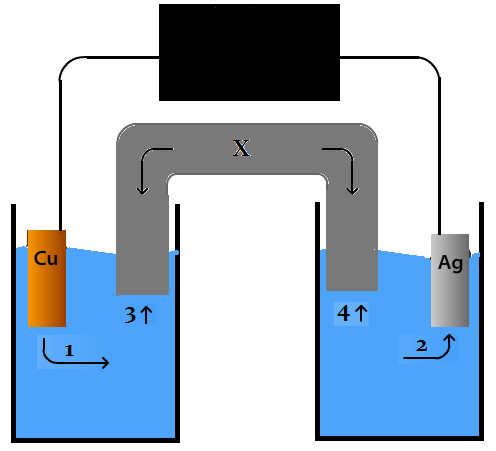Instead of silver, a scientist uses a strip of zinc in the opposite half cell from copper. Which of the following is true when comparing this new cell to the cell in the diagram?

The reduction potential of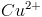is 0.34 volts. The reduction potential for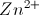is -0.76 volts.

The direction of electron flow is the same in both cells

Ions are no longer generated in the new cell

The cathode is the same in both cells

Energy can be produced in both cells

The direction of ion migration in the salt bridge is the same in both cells

Energy can be produced in both cells

Explanation:

In the new cell, energy can still be produced, but because zinc ions have a lower reduction potential than copper ions, the copper will be reduced and the direction of electron flow will be reversed, as compared to the cell with silver in which copper was oxidized.

### Example Question #2 : Electrochemistry

A student conducts an experiment for a chemistry class. The student wishes to explore power generation from different types of voltaic cells. He sets up three different cells, and then compares the amount of energy generated from each one.

One of his cells is shown below as an example. Both remaining cells follow the same layout.If there is a net production of copper ions in the half cell on the left as the reaction proceeds, which of the following must be true?

There is no net electron flow

Silver has a more positive reduction potential than copper

Copper is reduced in the reaction

Copper has a more positive reduction potential than silver

Silver is oxidized in the reaction

Silver has a more positive reduction potential than copper

Explanation:

If copper ions are generated as the voltaic cell functions, then the copper is being oxidized, and the silver must be reduced. Reduction and oxidation always occur together in a coupled reaction. This must also mean that the reduction potential for Ag is higher than the reduction potential for Cu.

### Example Question #3 : Electrochemistry

A student conducts an experiment for a chemistry class. The student wishes to explore power generation from different types of voltaic cells. He sets up three different cells, and then compares the amount of energy generated from each one.

One of his cells is shown below as an example. Both remaining cells follow the same layout.As the difference in the reduction potentials between two half cells increases, what happens to the Gibbs free energy of the reaction?

It becomes more positive

It will increase or decrease, depending on the species involved

It does not change, as thermodynamics is independent of reduction potential

It only changes with changes in temperature or pressure, and is independent of the chemical species involved

It becomes more negative

It becomes more negative

Explanation:

The reduction potential of a cell is directly related to the Gibbs free energy by the equation below.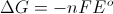As the reduction potential of a cell becomes more and more positive, the Gibbs free energy value becomes more and more negative.

### Example Question #1 : Electrochemistry

Imagine a galvanic cell which uses solid zinc and aqueous iron ions to produce a voltage.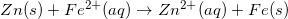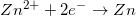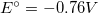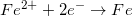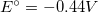What is the standard state cell potential for this reaction?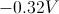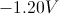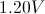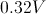Explanation:

Keep in mind that a galvanic cell will always have a positive voltage, so you can disregard the negative options. The half reactions show the voltage that will result if the element in question is reduced; however, an oxidation-reduction reaction will always have one element oxidized and another element reduced. In the equation shown, solid zinc (Zn) is oxidized, so the voltage of its half reaction is inverted to +0.76V. Next, you add the voltage of iron's reduction, resulting in the overall voltage of the galvanic cell.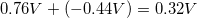### Example Question #5 : Electrochemistry

Imagine a galvanic cell which uses solid zinc and aqueous iron ions to produce a voltage.Suppose that this galvanic cell was converted into an electrolytic cell. Which of the following statements would be true?

The cell potential would be negative

Oxidation would take place at the cathode

The reaction is spontaneous

No electrons would flow from anode to cathode

The cell potential would be negative

Explanation:

An electrolytic cell is best thought of as a cell that requires an external power source in order to work. The reaction will go in the opposite direction of a galvanic cell, meaning that the cell potential will also be inversed and the reaction will be non-spontaneous. As a result, cell potential would be negative in an electrolytic cell.

### Example Question #11 : Electrochemistry

Imagine a galvanic cell which uses solid zinc and aqueous iron ions to produce a voltage.Assuming standard conditions, what is the free energy for the reaction?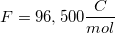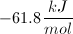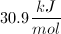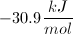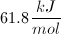Explanation:

Since the reaction is taking place under standard conditions, we can determine the free energy of the reaction by using the equation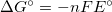.

n is the number of moles of electrons transferred in the balanced reaction, F is Faraday's constant, and Eo is the cell potential for the reaction.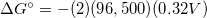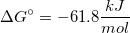### Example Question #12 : Electrochemistry

Determine the cell potential of the following reaction.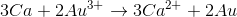Reduction potentials of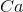and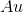cations are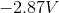and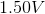, respectively.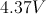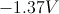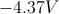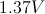Explanation:

In the given reaction calcium,, is oxidized (loses electrons) and gold,, is reduced (gains electrons).

We are only given the reduction potentials. The oxidation potential ofis the negative of the reduction potential: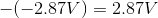.

Recall that only the moles of electrons must balance for these reactions, therefore no multiplication of the standard potentials is needed when balancing mole atoms; thus the cell potential is the sum of the calcium oxidation potential and the gold reduction potential.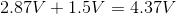### Example Question #13 : Electrochemistry

Consider the following half reactions and corresponding reduction potentials: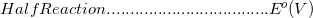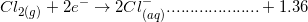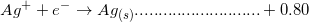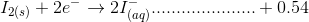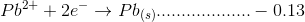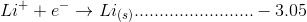Based on the above information, which substance can oxidize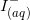to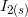?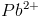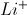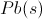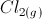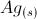Explanation:

The reduction potential of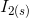is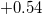, so the corresponding oxidation potential of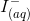is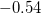.

For a substance to oxidizeto, it must have reduction potential greater than, so that the sum of the reduction potential of this compounds and the oxidation potential ofis positive.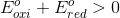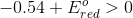The only choice that meets this requirement is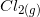.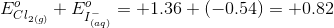### Example Question #1 : Half Reactions And Reduction Potential

A student conducts an experiment for a chemistry class. The student wishes to explore power generation from different types of voltaic cells. He sets up three different cells, and then compares the amount of energy generated from each one.

One of his cells is shown below as an example. Both remaining cells follow the same layout.In the voltaic cell drawn above, what is true about the species Ag?

The reduction potential foris 0.34. The reduction potential for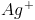0.80.

It is reduced and gains electrons

It is reduced and loses electrons

It is oxidized and loses electrons

It is oxidized and gains electons

It is neither reduced nor oxidized